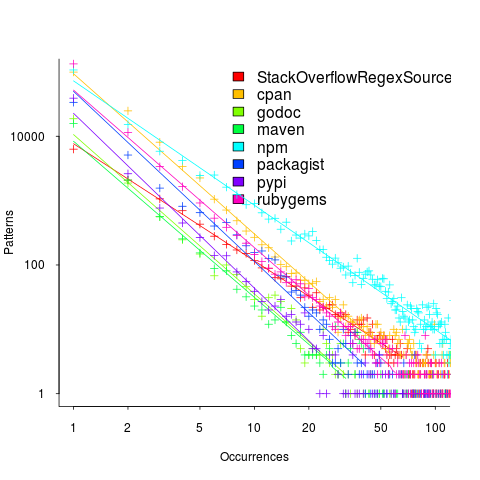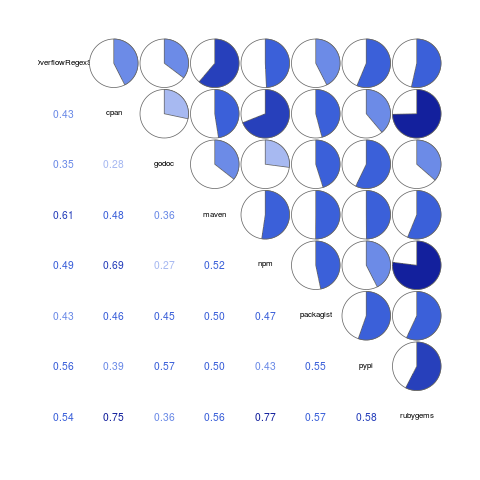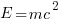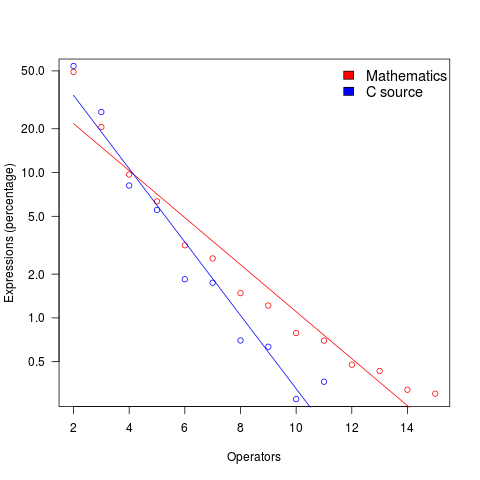## Patterns of regular expression usage: duplicate regexs

Regular expressions are widely used, but until recently they were rarely studied empirically (i.e., just theory research).

This week I discovered two groups studying regular expression usage in source code. The VTLeeLab has various papers analysing 500K distinct regular expressions, from programs written in eight languages and StackOverflow; Carl Chapman and Peipei Wang have been looking at testing of regular expressions, and also ran an interesting experiment (I will write about this when I have decoded the data).

Regular expressions are interesting, in that their use is likely to be purely driven by an application requirement; the use of an integer literals may be driven by internal housekeeping requirements. The number of times the same regular expression appears in source code provides an insight (I claim) into the number of times different programs are having to solve the same application problem.

The data made available by the VTLeeLab group provides lots of information about each distinct regular expression, but not a count of occurrences in source. My email request for count data received a reply from James Davis within the hourThe plot below (code+data; `crates.io` has not been included because the number of regexs extracted is much smaller than the other repos) shows the number of unique patterns (y-axis) against the number of identical occurrences of each unique pattern (x-axis), e.g., far left shows number of distinct patterns that occurred once, then the number of distinct patterns that each occur twice, etc; colors show the repositories (language) from which the source was obtained (to extract the regexs), and lines are fitted regression models of the form:, where:is driven by the total amount of source processed and the frequency of occurrence of regexs in source, andis the rate at which duplicates occur.So most patterns occur once, and a few patterns occur lots of times (there is a long tail off to the unplotted right).

The following table shows values offor the various repositories (languages):

```StackOverflow   cpan    godoc    maven    npm  packagist   pypi   rubygems
-1.8        -2.5     -2.5    -2.4    -1.9     -2.6     -2.7     -2.4
```

The lower (i.e., closer to zero) the value of, the more often the same regex will appear.

The values are in the region of -2.5, with two exceptions; why might StackOverflow and npm be different? I can imagine lots of duplicates on StackOverflow, but npm (I’m not really familiar with this package ecosystem).

I am pleased to see such good regression fits, and close power law exponents (I would have been happy with an exponential fit, or any other equation; I am interested in a consistent pattern across languages, not the pattern itself).

Some of the code is likely to be cloned, i.e., cut-and-pasted from a function in another package/program. Copy rates as high as 70% have been found. In this case, I don’t think cloned code matters. If a particular regex is needed, what difference does it make whether the code was cloned or written from scratch?

If the same regex appears in source because of the same application requirement, the number of reuses should be correlated across languages (unless different languages are being used to solve different kinds of problems). The plot below shows the correlation between number of occurrences of distinct regexs, for each pair of languages (or rather repos for particular languages; top left is StackOverflow).Why is there a mix of strong and weakly correlated pairs? Is it because similar application problems tend to be solved using different languages? Or perhaps there are different habits for cut-and-pasted source for developers using different repositories (which will cause some patterns to occur more often, but not others, and have an impact on correlation but not the regression fit).

There are lot of other interesting things that can be done with this data, when connected to the results of the analysis of distinct regexs, but these look like hard work, and I have a book to finish.

## Comparing expression usage in mathematics and C source

Why does a particular expression appear in source code?

One reason is that the expression is the coded form of a formula from the application domain, e.g.,.

Another reason is that the expression calculates an algorithm/housekeeping related address, or offset, to where a value of interest is held.

Most people (including me, many years ago) think that the majority of source code expressions relate to the application domain, in one-way or another.

Work on a compiler related optimizer, and you will soon learn the truth; most expressions are simple and calculate addresses/offsets. Optimizing compilers would not have much to do, if they only relied on expressions from the application domain (my numbers tool throws something up every now and again).

What are the characteristics of application domain expression?

I like to think of them as being complicated, but that’s because it used to be in my interest for them to be complicated (I used to work on optimizers, which have the potential to make big savings if things are complicated).

Measurements of expressions in scientific papers is needed, but who is going to be interested in measuring the characteristics of mathematical expressions appearing in papers? I’m interested, but not enough to do the work. Then, a few weeks ago I discovered: An Analysis of Mathematical Expressions Used in Practice, by Clare So; an analysis of 20,000 mathematical papers submitted to arXiv between 2000 and 2004.

The following discussion uses the measurements made for my C book, as the representative source code (I keep suggesting that detailed measurements of other languages is needed, but nobody has jumped in and made them, yet).

The table below shows percentage occurrence of operators in expressions. Minus is much more common than plus in mathematical expressions, the opposite of C source; the ‘popularity’ of the relational operators is also reversed.

```Operator  Mathematics   C source
=         0.39          3.08
-         0.35          0.19
+         0.24          0.38
<=        0.06          0.04
>         0.041         0.11
<         0.037         0.22
```

The most common single binary operator expression in mathematics is `n-1` (the data counts expressions using different variable names as different expressions; yes, `n` is the most popular variable name, and adding up other uses does not change relative frequency by much). In C source `var+int_constant` is around twice as common as `var-int_constant`

The plot below shows the percentage of expressions containing a given number of operators (I've made a big assumption about exactly what Clare So is counting; code+data). The operator count starts at two because that is where the count starts for the mathematics data. In C source, around 99% of expressions have less than two operators, so the simple case completely dominates.For expressions containing between two and five operators, frequency of occurrence is sort of about the same in mathematics and C, with C frequency decreasing more rapidly. The data disagrees with me again...